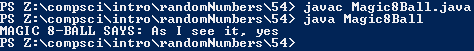# Assignemnt #54, Magic 8 Ball

## Code

```      import java.util.Random;

public class Magic8Ball
{
public static void main ( String[] args )
{
Random r = new Random();

int choice = 1 + r.nextInt(20);
String response = "";

if ( choice == 1 )
response = "It is certain";
else if ( choice == 2 )
response = "It is decidedly so";
else if ( choice == 3 )
response = "Without a doubt";
else if ( choice == 4 )
response = "Yes - definitely";
else if ( choice == 5 )
response = "You may rely on it";
else if ( choice == 6 )
response = "As I see it, yes";
else if ( choice == 7 )
response = "Most likely";
else if ( choice == 8 )
response = "Outlook good";
else if ( choice == 9 )
response = "Signs point to yes";
else if ( choice == 10 )
response = "Yes";
else if ( choice == 11 )
response = "Reply hazy, try again";
else if ( choice == 12 )
else if ( choice == 13 )
response = "Better not tell you now";
else if ( choice == 14 )
response = "Cannot predict now";
else if ( choice == 15 )
response = "Concentrate and ask again";
else if ( choice == 16 )
response = "Don't count on it";
else if ( choice == 17  )
response = "My reply is no";
else if ( choice == 18 )
response = "My sources say no";
else if ( choice == 19 )
response = "Outlook is not so good";
else if ( choice == 20 )
response = "Very doubtful";a
else
response = "8-BALL ERROR!";

System.out.println( "MAGIC 8-BALL SAYS: " + response );
}
}
```

### Picture of the output